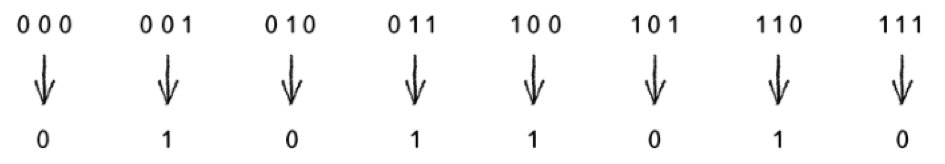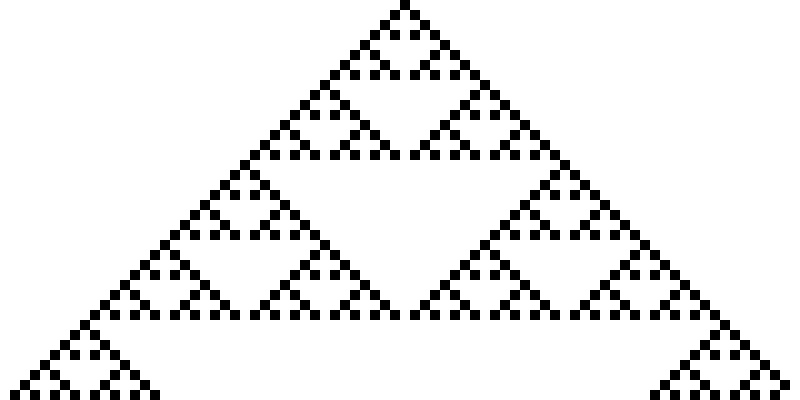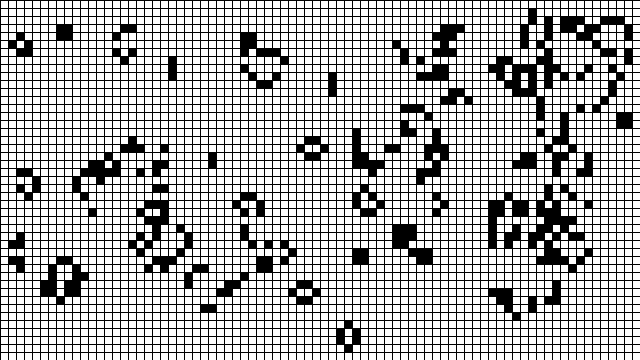# CS2D7 - Lab 9 - Cellular Automita

## Grids

Most cellular automata algorithms are done using a grid based system. As such, we need to create a grid! To make things easier, we've created a `Grid` class to make your life easier. Copy the below code and try it out! It can be set such that the dimensions of the grid can be modified, along with functions to set up an initial grid (both a randomised version and clear version) and to draw the grid based on two colours.

`class Grid {  private int xSize, ySize;  //Dimensions of the grid  private boolean[][] rawGrid;  //The raw data   Grid(int newXSize, int newYSize) {    xSize = newXSize;    ySize = newYSize;     rawGrid = new boolean[xSize][ySize];  } //Produce a cleared grid  public void clearGrid() {    for (int i = 0; i < xSize; i++) {      for (int j = 0; j < ySize; j++) {        rawGrid[i][j] = false;      }    }  }//Produce a randomised grid    public void randomiseGrid() {    for (int i = 0; i < xSize; i++) {      for (int j = 0; j < ySize; j++) {        if (round(random(0,1)) == 0) {          rawGrid[i][j] = false;        } else {          rawGrid[i][j] = true;        }      }    }  } //Display the grid  public void display(color trueColour, color falseColour) {    for (int i = 0; i < xSize; i++) {      for (int j = 0; j < ySize; j++) {        noStroke();  //Get rid of the outline        //Colour the cell appropriately        if (rawGrid[i][j]) {          fill(trueColour);        } else {          fill(falseColour);        }        //Draw the cell        rect(i*(int)(width/xSize),            j*(int)(height/ySize),            (i+1)*(int)(width/xSize),            (j+1)*(int)(height/ySize)        );       }    }  }   public void setPos(int x, int y, boolean value) {rawGrid[x][y] = value;}  public boolean getPos(int x, int y) {return rawGrid[x][y];} }Grid grid;void setup() {  size(800, 800);  grid = new Grid(100, 100);  grid.randomiseGrid();}void draw() {//True is black (0), False is white (255)  grid.display(color(0), color(255));}`

## Wolfram's Elementary CA

The simplest CA has cells that live on a one-dimensional grid: a line of cells. Each cell is either on (True) or off (False). This is the initial state (generation 0).

The neighbourhood for any given cell is the cell itself and its two neighbours: one to the left and one to the right.

Each time we run draw, we compute the states for all cells at the next generation based on a set of rules.

Once we have defined all the possible neighbourhoods, we need to define an outcome (new state value: False or True) for each neighbourhood configuration.

For example:The above ruleset is commonly referred to as “Rule 90” because if you convert the binary sequence—01011010—to a decimal number, you’ll get the integer 90.

The standard Wolfram model is to start generation 0 with all cells having a state of 0 except for the middle cell, which should have a state of 1.

To calculate the cell state in the next generation we need to:

1. Take a look at the neighbourhood states: left, middle, right.

2. Look up the new value for the cell state according to some ruleset.

3. Set the cell’s state to that new value.

4. Display the array in a visual form

## Exercise 1

Create a 2-dimensional visualisation of a 1-dimensional CA starting with with all cells having a state of 0 except for the middle cell and using Rule 90. What happens if you apply the same rule but initialise the array such that each cell has a random state?## Game of Life

The Game of Life is a very famous cellular automaton devised by the British mathematician John Conway in 1970.

The game is usually played on a 2-dimensional grid. Cells are either alive (black) or dead (white). After an initial pattern is set, subsequent generations are created by applying a set of rules to each cell and its surrounding 8 neighbours:

• Any live cell with fewer than two live neighbours dies - loneliness
• Any live cell with more than three live neighbours dies - overcrowding
• Any dead cell with exactly three live neighbours becomes a live cell - reproduction

To calculate the cell state in the next generation we need to:

1. Take a look at the neighbourhood states: The 8 surrounding cells.

2. Look up the new value for the cell state according to the ruleset.

3. Set the cell’s state to that new value.

4. Display the 2D array in a visual form

## Exercise 2

Create a visualisation of the Game of Life starting with a randomly initialised grid and applying the rules above.## Bonus Exercise 1

Experiment with different rules with Wolfram's Elementary CA (list of some rules can be found here).

## Bonus Exercise 2

Rewrite the rules of Game Of Life such that if the cell has four or more alive neighbours, it has a 80% chance of dying (over population) and if the cell has one or fewer alive neighbours, it has a 60% chance of dying (loneliness).